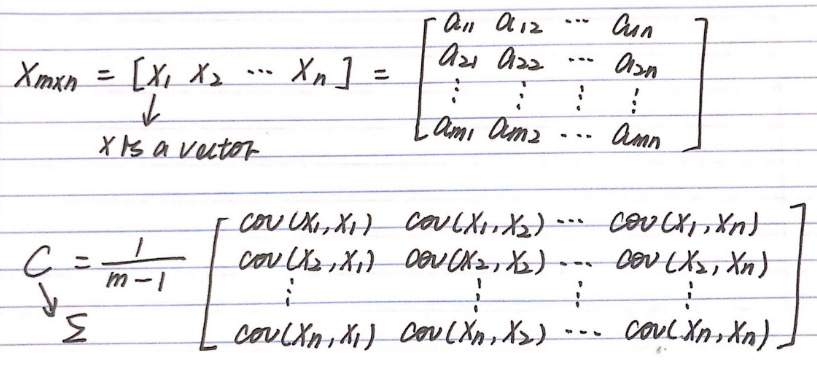# Yitong Ren - «The significance and applications of covariance matrix» (2019-05-01)

Covariance matrix is one simple and useful math concept that is widely applied in financial engineering, econometrics as well as machine learning.

Covariance measures how much two random variables vary together in a population.

When the population contains higher dimensions or more random variables, a matrix is used to describe the relationship between different dimensions.

In a more easy-to-understand way, covariance matrix is to define the relationship in the entire dimensions as the relationships between every two random variables.## Use Case 1: Stochastic Modeling

The most important feature of covariance matrix is that it is positive semi-definite, which brings about Cholesky decomposition.

In a nutshell, Cholesky decomposition is to decompose a positive definite matrix into the product of a lower triangular matrix and its transpose.

In practice, people use it to generate correlated random variables by multiplying the lower triangular from decomposing covariance matrix by standard normals.

towardsdatascience.com/the-significance-and-applications-of-covariance-matrix-d021c17bce82